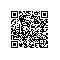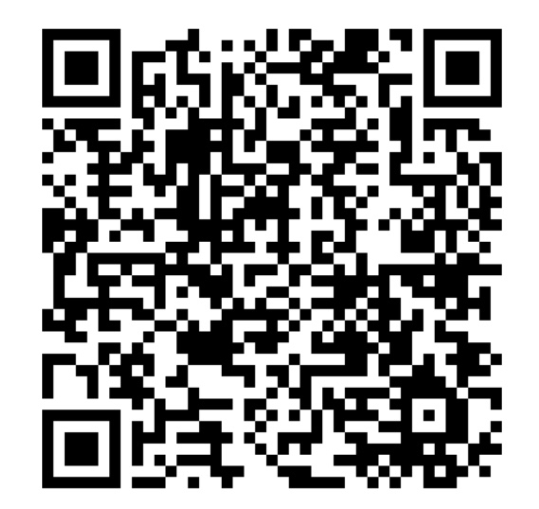# 数值和字符串互相转换

今天看书看到了strintstream，感觉用起来很方便，尤其是将数值转换为字符串的时候使用stringstream，可以达到非常美妙的效果。对比前面我的一篇文章--如何将数字转换为字符串，使用#的方法，使用stringstream也是一种很好的选择。
废话不多说，直接看代码吧。
main.cpp文件:#include <iostream>#include <sstream>using namespace std;int main(){stringstream ss;    //流输出ss << "there are " << 100 << " students.";cout << ss.str() << endl;int intNumber = 10;    //int型ss.str("");ss << intNumber;cout << ss.str() << endl;float floatNumber = 3.14159f;  //float型ss.str("");ss << floatNumber;cout << ss.str() << endl;int hexNumber = 16;        //16进制形式转换为字符串ss.str("");ss << showbase << hex << hexNumber;cout << ss.str() << endl;return 0;}
输出结果如下：
there are 100 students.
10
3.14159
0x10
可以看出使用stringstream比较使用#的好处是可以格式化数字，以多种形式（比如十六进制）格式化，代码也比较简单、清晰。

同样，可以使用stringstream将字符串转换为数值：#include <iostream>#include <sstream>using namespace std;template<class T>T strToNum(const string& str)  //字符串转换为数值函数{stringstream ss(str);T temp;ss >> temp;if ( ss.fail() ) {string excep = "Unable to format ";excep += str;throw (excep);}return temp;}int main(){try {string toBeFormat = "7";int num1 = strToNum<int>(toBeFormat);cout << num1 << endl;toBeFormat = "3.14159";double num2 = strToNum<double>(toBeFormat);cout << num2 << endl;toBeFormat = "abc";int num3 = strToNum<int>(toBeFormat);cout << num3 << endl;}catch (string& e) {cerr << "exception:" << e << endl;}return 0;}

这样就解决了我们在程序中经常遇到的字符串到数值的转换问题。

本文转自panpan3210 51CTO博客，原文链接：http://blog.51cto.com/panpan/107732，如需转载请自行联系原作者使用钉钉扫一扫加入圈子
+ 订阅